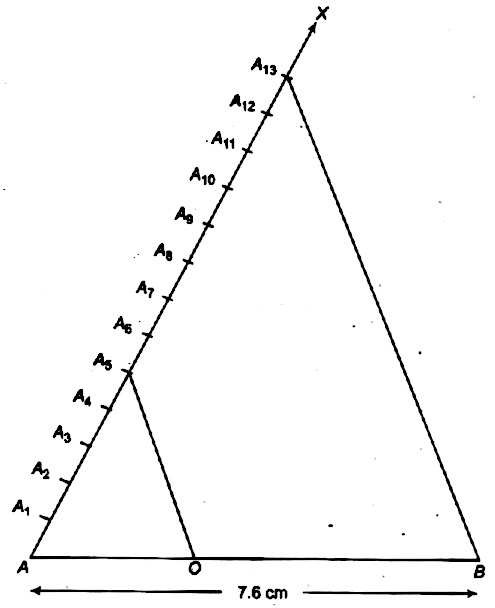Home/Class 10 Math Chapter List/10. Constructions/

# Draw a line segment of length (7.6;cm) and divide it in the ratio (5:8.)Measure the two parts.

## QuestionMathClass 10

Draw a line segment of length $$7.6\;cm$$ and divide it in the ratio $$5:8.$$Measure the two parts.

see the solution below
4.64.6## Solution

Steps of construction·
1.Draw a line segment$$AB=7.6\;cm$$
2.Draw a ray $$AX,$$making an acute $$\angle BAX$$.
3. Along$$AX,$$. mark$$5+8=13$$points $$A_{1},A_{2},A_{3},A_{4},\cdots ,A_{12},A_{13}$$such that
$$AA_{1}=A_{1}A_{2}=A_{2}A_{3}=A_{3}A_{4}=A_{4}A_{5}=A_{5}A_{6}=A_{6}A_{7}$$$$=A_{7}A_{8}=A_{8}A_{9}=A_{9}A_{10}=A_{10}A_{11}=A_{11}A_{12}=A_{12}A_{13}$$
4. Join$$A_{13}B.$$
5. From$$A_{5}$$draw$$A_{5}O|| A_{13}B$$ meeting $$AB$$ at $$O$$. [By making an angle equal to $$\angle AA_{13}B]$$
Then, $$O$$ is the point on AB which divides it in the ratio $$5:8$$.
So,$$AO:OB=5:8$$Justification
Let $$AA_{1}=A_{1}A_{2}=A_{2}A_{3}=A_{3}A_{4}=A_{4}A_{5}$$
$$=A_{5}A_{6}=A_{5}A_{7}=\cdots =A_{12}A_{13}=x$$
$$\Delta ABA_{13},$$we have
$$A_{5}O|| A_{13}B$$
$$\therefore \frac {AO}{OB}=\frac {AA_{5}}{A_{5}A_{13}}=\frac {5x}{8x}=\frac {5}{8}$$      (By basic proportionality theorem)
Hence,$$AO:OB=5:8$$
On measuring, we find that$$AO=2.9\;cm$$
and $$OB=4.7\;cm$$